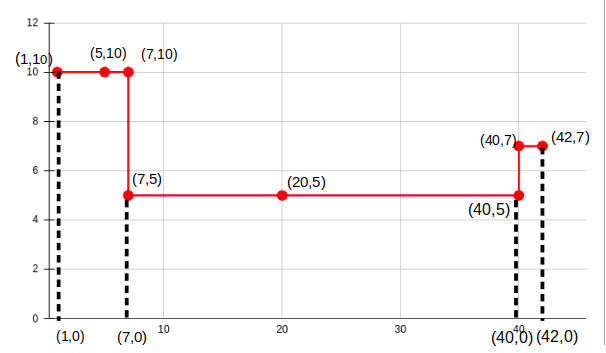Problem title
Difficulty
Avg time to solve

Number of GP sequence
Moderate
10 mins
Perfect Number
Easy
10 mins
Form the Biggest Number
Moderate
25 mins
Check Square
Moderate
10 mins
Sorted Subsequence of Size 3
Moderate
15 mins
Remove maximum edges
Easy
15 mins
Angle Between Hour Hand And Minute Hand
Easy
15 mins
3 Divisors
Moderate
25 mins
Maximum Length of Chain
Moderate
15 mins
Distinct Paths
Moderate
20 mins2

# Area Under Curve

Difficulty: MEDIUM
Avg. time to solve
30 min
Success Rate
60%

Problem Statement

#### The area under the curve along the X-axis is defined as the total area covered by the curve and the line Y = 0. See the sample explanation figure below for further clarification.

##### Note
``````1. The coordinates will be given in non-descending order of X coordinate from start to end of the linked list.

2. All the coordinates will be pairwise distinct i.e there are no two coordinates (X1, Y1) and (X2, Y2) such that X1 =  X2 and Y1 = Y2 and there will be an edge between every two consecutive coordinates.

3. The first coordinate and the last coordinate in the input can be assumed as the starting point and the ending point of the curve respectively and there will not be an edge between these two coordinates.
``````
##### Input Format:
``````The first line contains a single integer T denoting the number of test cases.

The first and the only line of each test case will contain 2 * N +1  linked list elements (separated by space and terminated by -1), where 'N' is the total number of coordinates.
Each node consists of two parts (X coordinate and Y coordinate).
``````
##### Output Format:
``````For each test case, print a single integer denoting the total area under the given curve.
``````
##### Note:
``````You do not need to print anything, it has already been taken care of. Just implement the given function and return the answer.
``````
##### Constraints:
``````1 <= T <= 10
1 <= N <= 10^4
-10^4 <= X, Y <= 10^4

Time Limit: 1 sec
``````
##### Sample Input 1:
``````2
1 10 5 10 7 10 7 5 20 5 40 5 40 7 42 7 -1
0 0 -1
``````
##### Sample Output 1:
``````239
0
``````
##### Explanation For Sample Input 1:
``````For the first test:

The curve can be represented on a X - Y plane as follows:
````````````Clearly we can divide the area under this curve into 3 rectangles of dimensions:
6 X 10 , 33 X 5 and 2 X 7. Thus the total area is 6 * 10 + 33 * 5 + 2* 7 = 239.

For the second test:
As there is only one point in the curve and which is the origin the area here will be zero.
``````
##### Sample Input 2:
``````2
2 4 2 4 5 4 -1
4 5 2 3 -1
``````
##### Sample Output 2:
``````12
0
``````Console